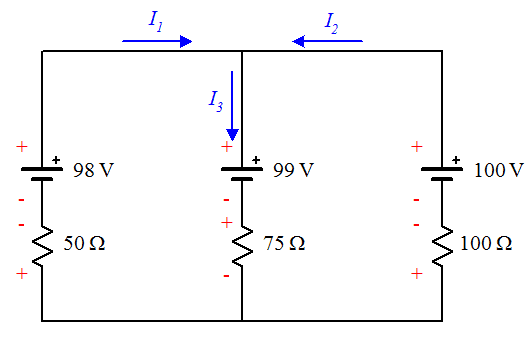# Finding currents using the junction and loop rules

## Homework Statementi1+i2-i3=0
50i1+75i3=1
100i2-75i3=1

## The Attempt at a Solution

matrix A=1 1 -1
0 100 -75[/B]
50 0 75
matrix B= 0
1
1

i1= -6ma
i2= 23ma
i3= 17ma

gneill
Mentor
Your diagram does not show the 20 Ω resistor mentioned in the problem statement. Nor does is show points a and b.

Are you sure that this diagram fits the described problem?

Sorry, we were told to omit that line.

gneill
Mentor
Check the signs of the terms in your KVL equations. Can you show some detail in your solution to the simultaneous equations? Are you using some software to solve them? Typing out a matrix doesn't tell us anything unless by some strange coincidence we happen to know what software you have and we have it too.

I am using a TI 83+. Matrix A is a 3x3 and matrix B is a 3x1. A^-1*B. I have checked my signs many times so if they are wrong I am not seeing it.

gneill
Mentor
Write out your KVL equations again without combining the voltage terms or transposing the voltage terms to the RHS of the equation (so the RHS should be a zero). Say which direction you are "walking" around the loop and whether you are counting potential drops as positive or negative quantities.

-50i1-75i3-1=0
100i2+75i3-1=0
i1+i2-i3=0

We were taught to place charges at each side of each component and the charge on the side you are exiting is the sign you use for your equation. The stuff I see online is a little different in terms of drops and gains. Not sure which to use?

gneill
Mentor
Okay. Those equations look correct. What values do you find with those equations?

Your circuit diagram is confusing because the potential drops that you've indicated for the resistors don't appear to obey the chosen current directions. For example, I1 is flowing upwards through the 50 Ω resistor but you marked its potential change as higher where the current exits the resistor (at its top).

I see what you're saying would this be correct:
-50i1+75i3-1=0
100i2-75i3-1=0
i1-i2-i3=0

i1=-15.64
i2=-7.8
i3=-7.8

gneill
Mentor
I see what you're saying would this be correct:
-50i1+75i3-1=0
100i2-75i3-1=0
i1-i2-i3=0
No, your last set of equations were okay.

Here's your circuit re-drawn with appropriate potential changes indicated:If you do a KVL walk clockwise around the loop on the left starting from the bottom left corner you encounter:

##-50 I_1 + 98 - 99 - 75 I_3 = 0##

##-50 I_1 - 75 I_3 - 1 = 0##

Can you do the same for the second loop?

75i3+99-100+100i2=0
100i2+75i3-1=0

Not sure why the 75 ohm resistor doesn't have the signs opposite what you show. Wouldn't the top side be negative from the 99v source above it?

gneill
Mentor
75i3+99-100+100i2=0
100i2+75i3-1=0
Correct.
Not sure why the 75 ohm resistor doesn't have the signs opposite what you show. Wouldn't the top side be negative from the 99v source above it?
Look at the direction that you've chosen for I3. It flows down through the 75 Ω resistor, hence the potential drop in that direction.

Ok. Now I get it. My current direction determines polarity!

I get:
i1=-15ma
i2=12ma
i3=-3ma

Still not the same answer instructor gets.

gneill
Mentor
Your results are correct for the circuit given. If your professor obtained different results from the same circuit then his results are incorrect.

Gee, there's a shocker! Thanks for your help! Off to take the test!

So I assume with a voltage source the polarity remains as whatever the source is? Meaning the current direction does not determine polarity through a source?

gneill
Mentor
So I assume with a voltage source the polarity remains as whatever the source is? Meaning the current direction does not determine polarity through a source?
Correct. A voltage source will produce or absorb any amount of current to maintain its specified potential difference.